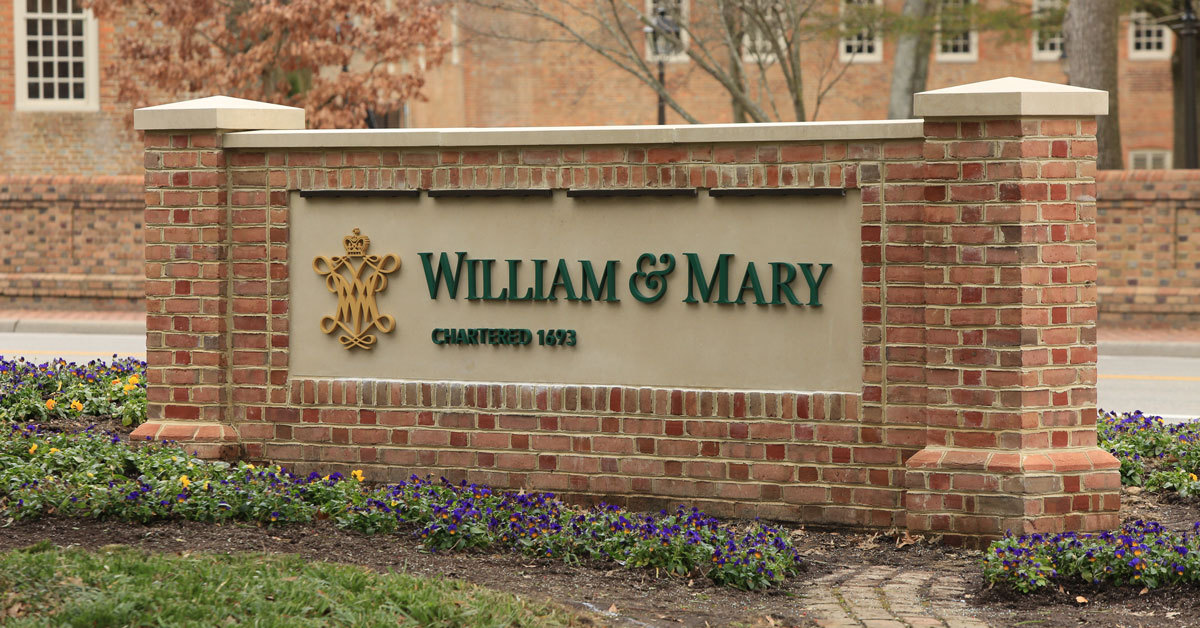Latest about COVID-19 and the Path Forward for fall.# Matrix problems in quantum information science

Quantum information science is an active research area,
and there are interesting problems suitable for undergraduate
research. Here are a few of them.
1)  Decomposition of quantum gates.

We try to decompose a unitary matrix as the product
of simple unitary matrices so that they can be easily
implemented. One may see the pdf file of Li's presentation
and the arXiv papers
2)  Interpolation problems in quantum information science.
Quantum states are represented as density matrices,
i.e., positive semi-definite matrices with trace 1.
A general quantum operation has the form
T(X) = F_1XF_1* + .... + F_rXF_r*
for some suitable choices of m x n matrices F_1, ..., F_r
such that F_1F_1* + .... + F_rF_r* = I.
Suppose A_1, ..., A_k are nxn density matrices
and B_1, ..., B_k are mxm density matrices.
We would like to know whether there exists a quantum
operation of the above form such that
T(A_i) = B_i   for i = 1, ..., k.
One may see the arXiv papers
for more background.